# Newton's 3 Laws of Motion: Force, Mass and Acceleration

Eugene is a qualified control/instrumentation engineer Bsc (Eng) and has worked as a developer of electronics & software for SCADA systems.

Mechanics is a branch of physics which deals with forces, mass, and motion.
In this second part of a two part tutorial, you'll learn the absolute basics!

In the first tutorial, we learned about forces. In this guide we cover:

• Newton's three laws of motion and how an object behaves when a force is applied
• Action and reaction
• Friction
• Kinematics equations of motion
• Work done and kinetic energy
• Momentum of a body
• Moments, couples and torque
• Angular velocity and power

## What Are Newton's Three Laws of Motion?

In the 17th century, the mathematician and scientist Isaac Newton came up with three laws of classical mechanics concerning motion of bodies in the Universe. These laws describe the relationship between the motion of an object and the forces acting on it.

## 1. Newton's First Law of Motion: Rest or Uniform Motion

Basically, this means that if for instance a ball is lying on the ground, it will stay there. If you kick it into the air, it will keep moving. If there was no gravity, it would go on for ever. However, the external force, in this case, is gravity which causes the ball to follow a curve, reach a max altitude and fall back to the ground. Friction from air also slows it down.

Another example is if you put your foot down on the gas and your car accelerates and reaches top speed. When you take your foot off the gas, the car slows down, The reason for this is that friction at the wheels and friction from the air surrounding the vehicle (known as drag) causes it to slow down. If these forces were magically removed, the car would stay moving forever.

### Body at Rest

There are two scenarios:

Scenario 1: No forces acting on the body

In the example of a body floating in space, where it isn't influenced by gravity, it will stay in this position at rest forever. In reality of course, it will always be influenced by gravity, even at huge distances, although the effect may be tiny.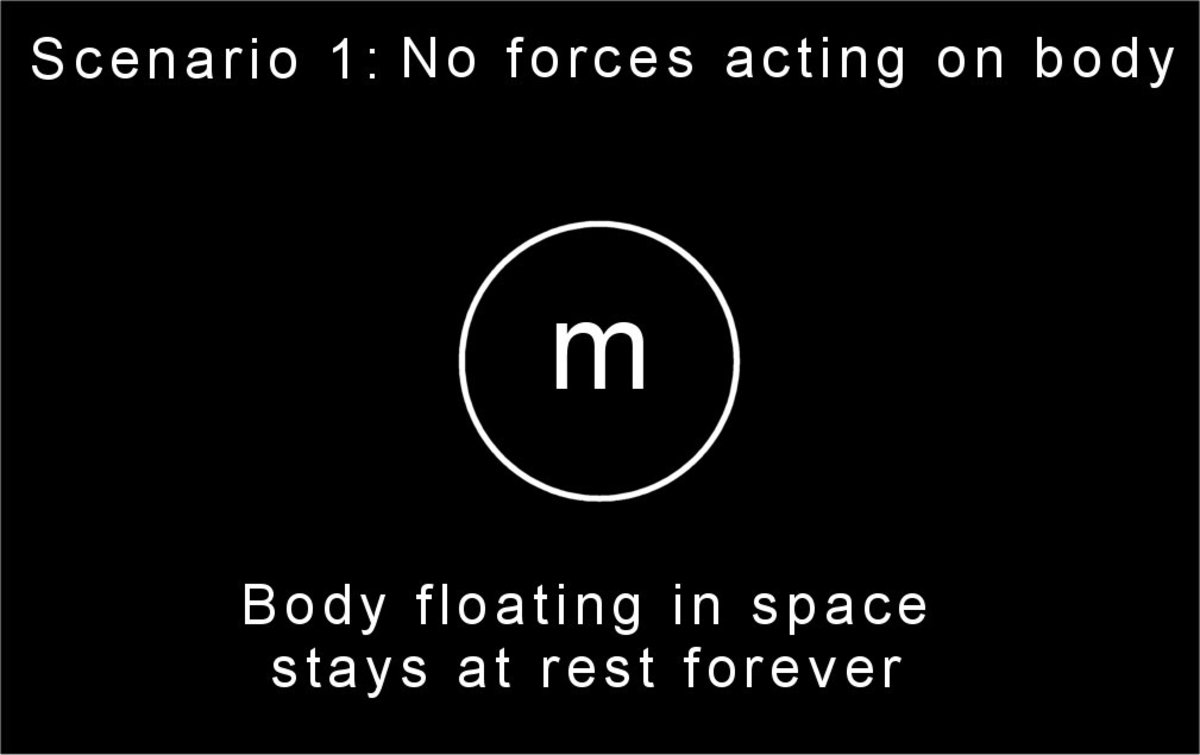No forces act on the body, so it stays at rest.© Eugene Brennan

Scenario 2: No net forces acting on the body.

If forces cancel out, there's no net force acting on a body and again it stays at rest. An example is a weight suspended from a rope.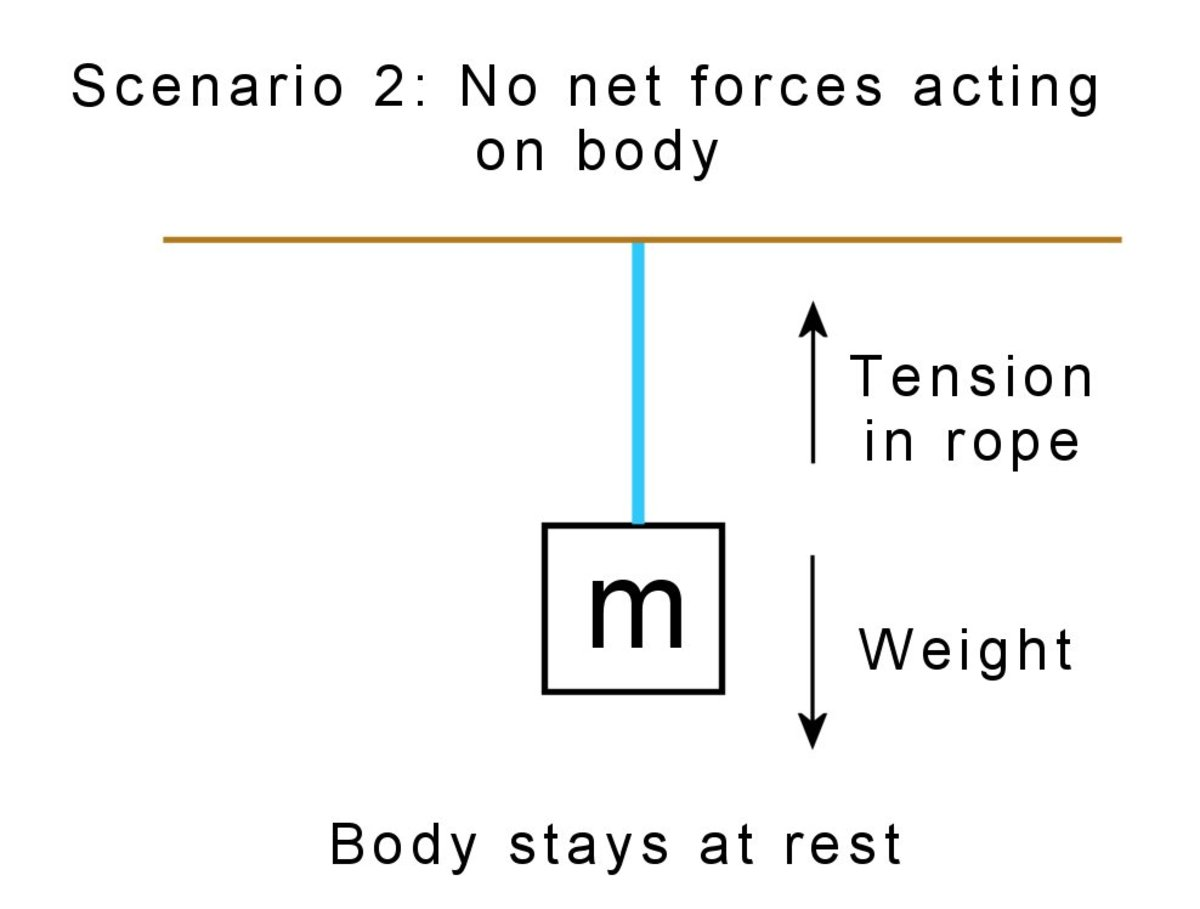Forces cancel out and since there's no net force, the body stays at rest.© Eugene Brennan
Scroll to Continue

## Read More From Owlcation

### Body in motion

When a body is acted on by a net force, it accelerates and reaches a certain velocity. When that force is removed, it continues to travel at that velocity, unless another force acts on it.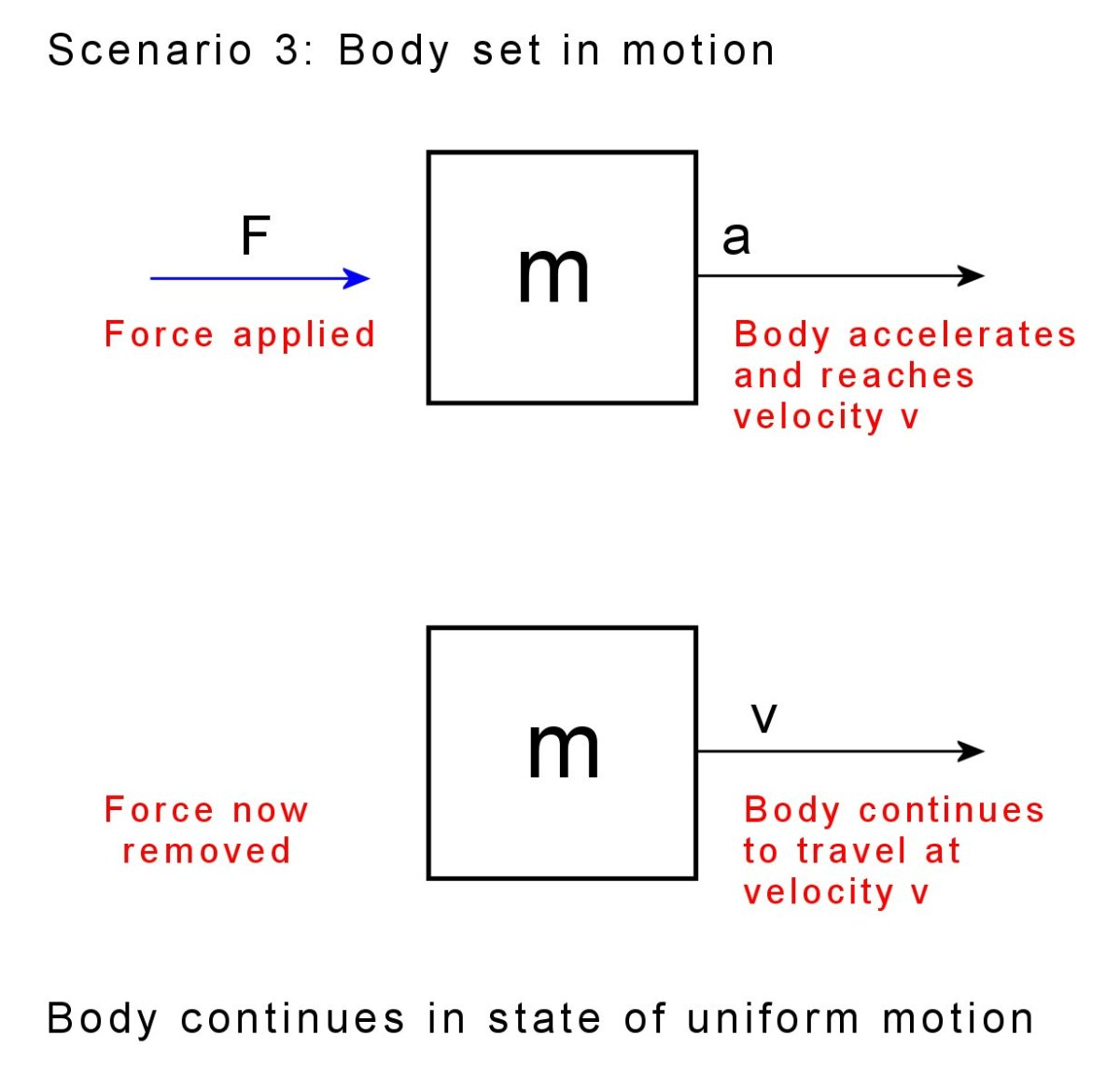Once a body is accelerated by a force, it continues in a state of uniform motion, unless another force acts on it.© Eugene Brennan

## 2. Newton's Second Law of Motion: Force and Acceleration

This means that if you have an object and you push it, the acceleration is greater for a greater force. So for example a 400 horse power engine in a sports car is going to create loads of thrust and accelerate the car to top speed rapidly.

If F is the force in Newtons (N)

m is the mass in kilos (kg)

and a is the acceleration

Then,

F = ma

Acceleration is measured in metres per second per second (or metres per second squared, abbreviated to m/s2)

We can also rearrange the equation so

a = F / m

So for larger masses, acceleration is smaller for the same force. Imagine in the example above that the sports car engine was placed into a heavy train locomotive and could drive the wheels. Because the mass of the locomotive is so large, the force creates much lower acceleration and the locomotive takes much longer to reach top speed.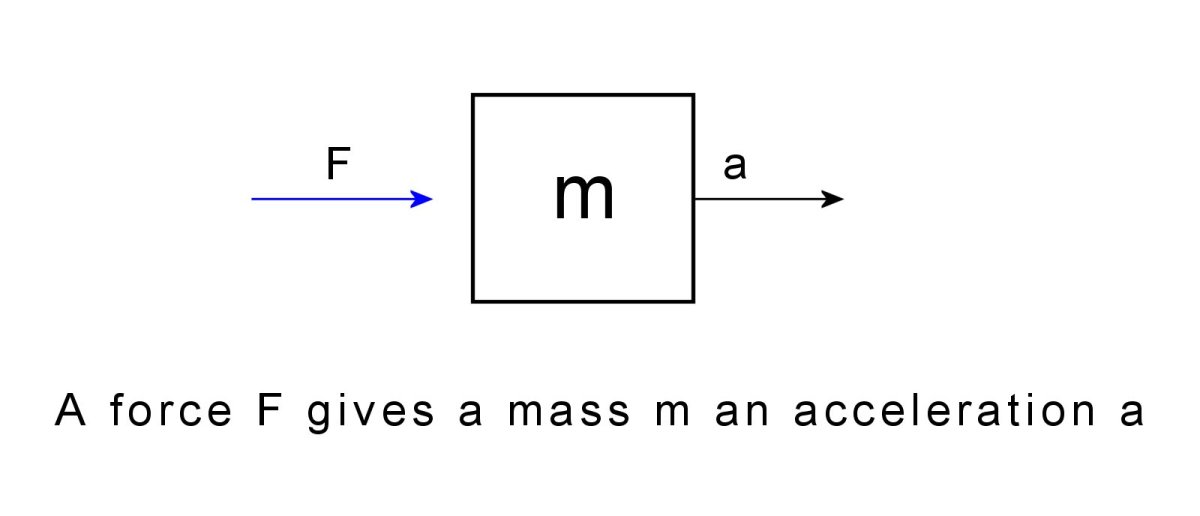A force accelerates an object with a mass m© Eugene Brennan

Example: A force of 10 newtons is applied to a mass of 2 kilos. What is the acceleration?

F = ma

So a = F / m = 10 / 2 = 5 m/s2

The velocity increases by 5 m/s every second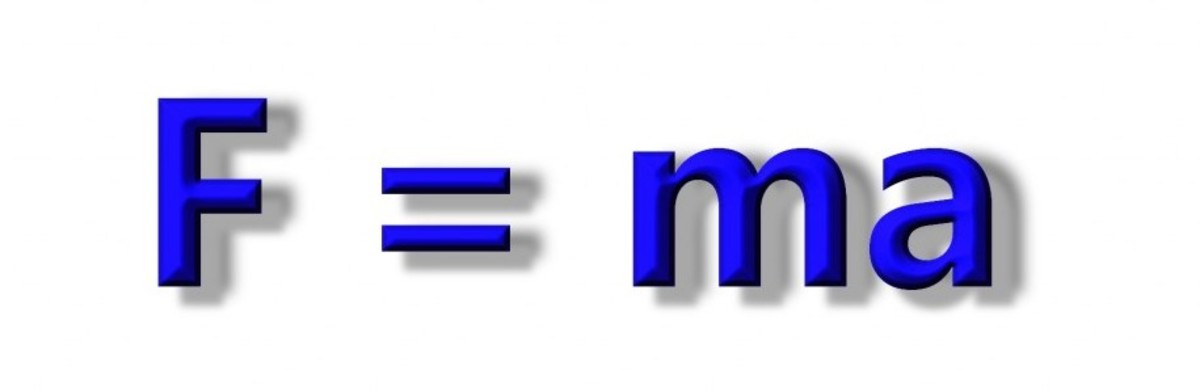Force = mass multiplied by acceleration. F = ma© Eugene Brennan

### Weight as a Force

In this case, the acceleration is g, and is known as the acceleration due to gravity.
g is approximately 9.81 m/s2 in the SI system of units.

Again F = ma

So if the force F is replaced by a variable for weight we choose to be W, then substituting for F and a gives:

W = ma = mg

Example: What is the weight of a 10 kg mass?

W = mg = 10 x 9.81 = 98.1 newtons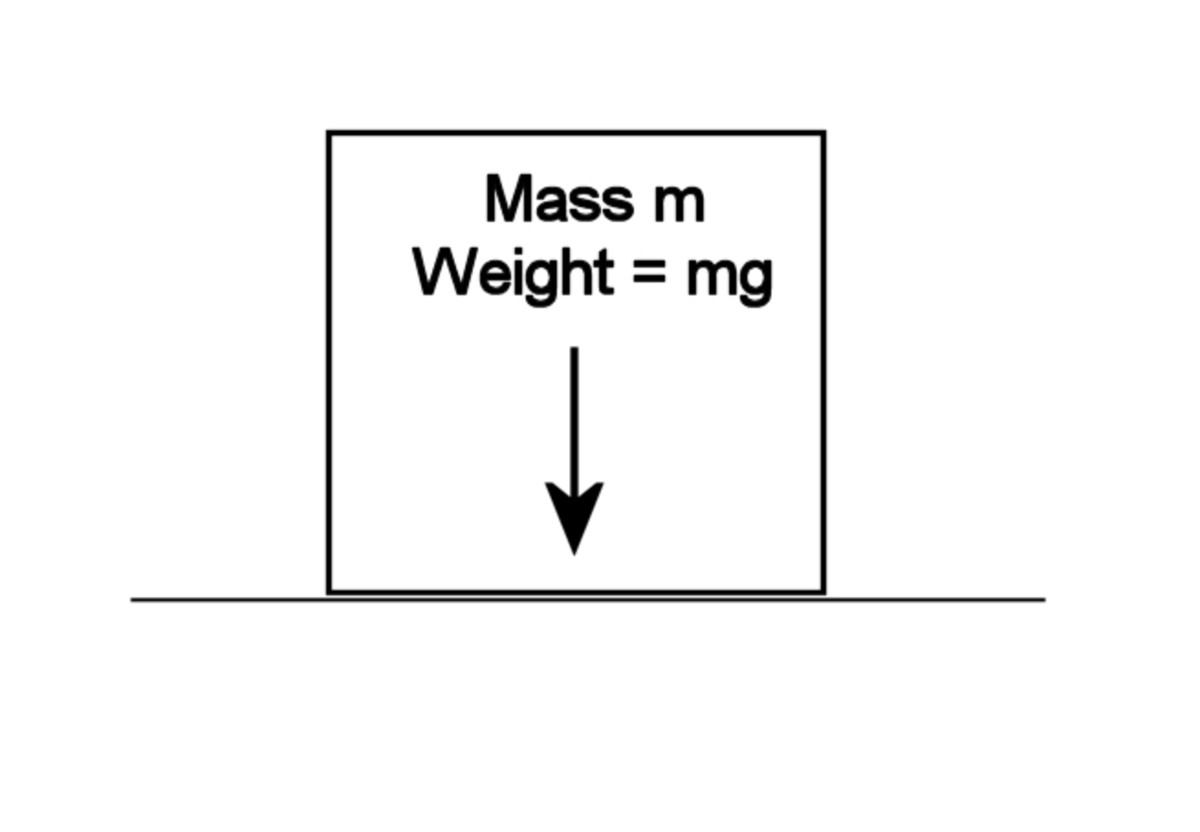Weight is the force on a mass due to gravity. The weight of a body of mass m equals mg, where g is the acceleration due to gravity© Eugene Brennan

## 3. Newton's Third Law of Motion: Action and Reaction

This means that when a force is exerted on an object, the object pushes back.

Some examples:

• When you push on a spring, the spring exerts a force back on your hand. If you push against a wall, the wall pushes back.
• When you stand on the ground, the ground supports you and pushes back up. If you try to stand on water, the water cannot exert enough force and you sink.
• Foundations of buildings must be able to support the weight of the construction. Columns, arches, trusses and suspension cables of bridges must exert enough reactive compressive or tensile force to support the weight of the bridge and what it carries.
• When you try to slide a heavy piece of furniture along the floor, friction opposes your effort and makes it difficult to slide the object

## What is Dry Friction or "Stiction"?

As we saw above, friction is an example of a force. When you attempt to slide a piece of furniture along a floor, friction opposes your effort and makes things more difficult. Friction is an example of a reactive force, and doesn't exist until you push the object (which is the active force). Initially, the reaction balances the applied force i.e. your effort pushing the furniture, and there is no movement. Eventually, as you push harder, the friction force reaches a maximum, known as the limiting force of friction. Once this value is exceeded by the applied force, the furniture will start to slide and accelerate. The friction force is still pushing back and this is what makes it so difficult to continue to slide the object. This is why wheels, bearings, and lubrication come in useful as they reduce friction between surfaces, and replace it by friction at an axle and leverage to overcome this friction. Friction is still necessary to stop a wheel sliding, but it doesn't oppose motion. Friction is detrimental as it can cause overheating and wear in machines resulting in premature wear. So engine oil is important in vehicles and other machines, and moving parts need to be lubricated.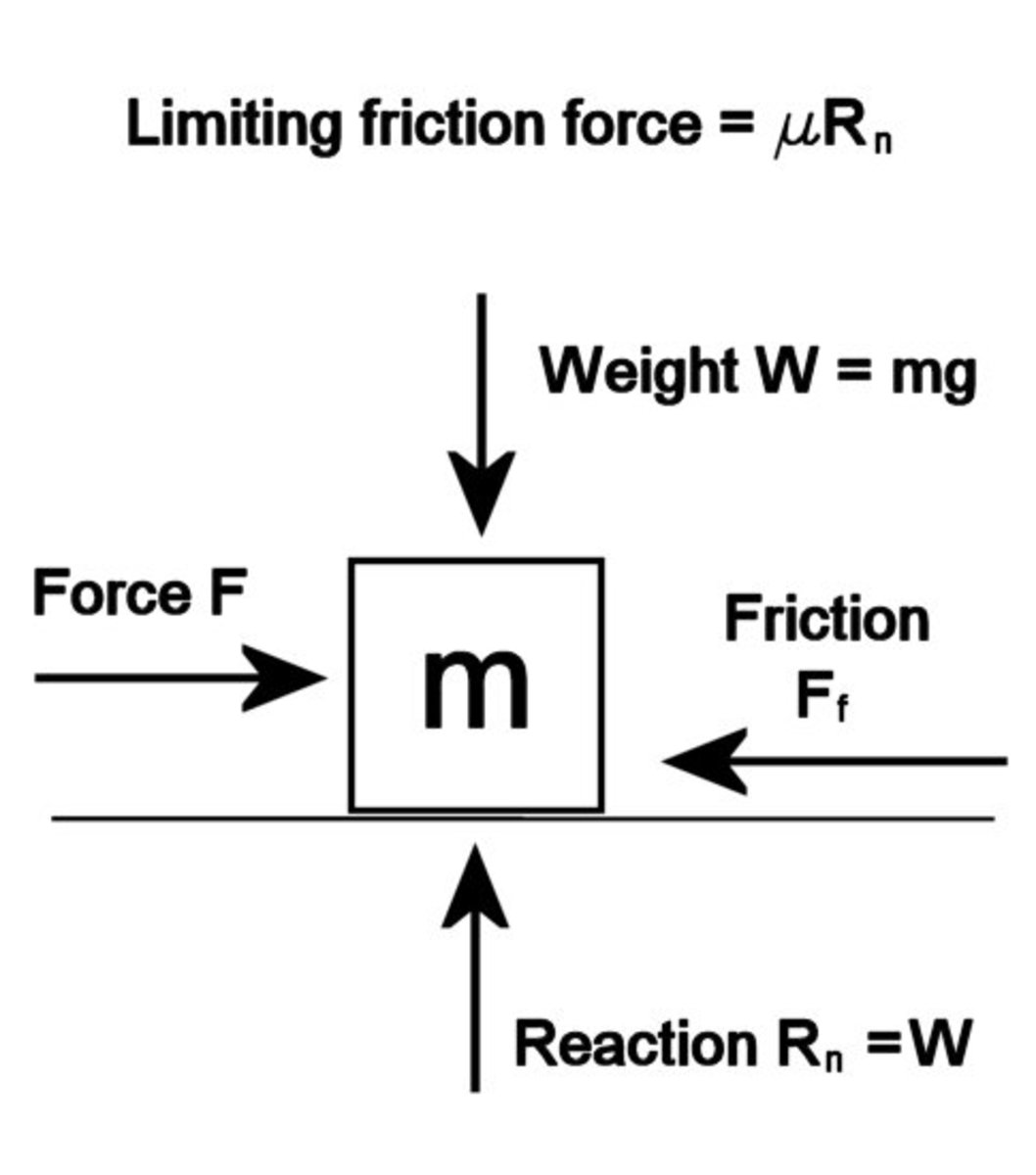Forces acting on a mass when a force attempts to slide it along a surface. When the mass is just about to slide, the friction force Ff reaches a maximum value μRn© Eugene Brennan

### Dry static friction, also known as stiction (see above diagram)

If F is the applied force on a body

The mass of the body is m

Weight of the body is W = mg

μs is the coefficient of friction (low μ means the surfaces are slippery)

and Rn is the normal reaction. (the reaction force acting upwards at right angles to the surface due to the weight of the object acting downwards)

If the surface is horizontal, then

Reaction Rn = Weight W

Then

limiting friction force is Ff = μsRn = μsW = μsmg

Remember this is the limiting force of friction just before sliding takes place. Before that, the friction force equals the applied force F trying to slide the surfaces along each other, and can be anything from 0 up to μRn.

So the limiting friction is proportional to the weight of an object. This is intuitive since it is harder to get a heavy object sliding on a specific surface than a light object. The coefficient of friction μ depends on the surface. "Slippery" materials such as wet ice and Teflon have a low μ. Rough concrete and rubber have a high μ. Notice also that the limiting friction force is independent of the area of contact between surfaces (not always true in practice)

### Kinetic Friction

Once an object starts to move, the opposing friction force becomes less than the applied force. The friction coefficient in this case is μk.

## What Are Newton's Equations of Motion? (Kinematics Equations)

There are three basic equations which can be used to work out the distance travelled, time taken and final velocity of an accelerated object.

First let's pick some variable names:

u is the initial velocity

v is the final velocity

s is the distance covered

t is the time taken

and a is the acceleration produced by force F

As long as the force is applied and there are no other forces, the velocity u increases uniformly (linearly) to v after time t.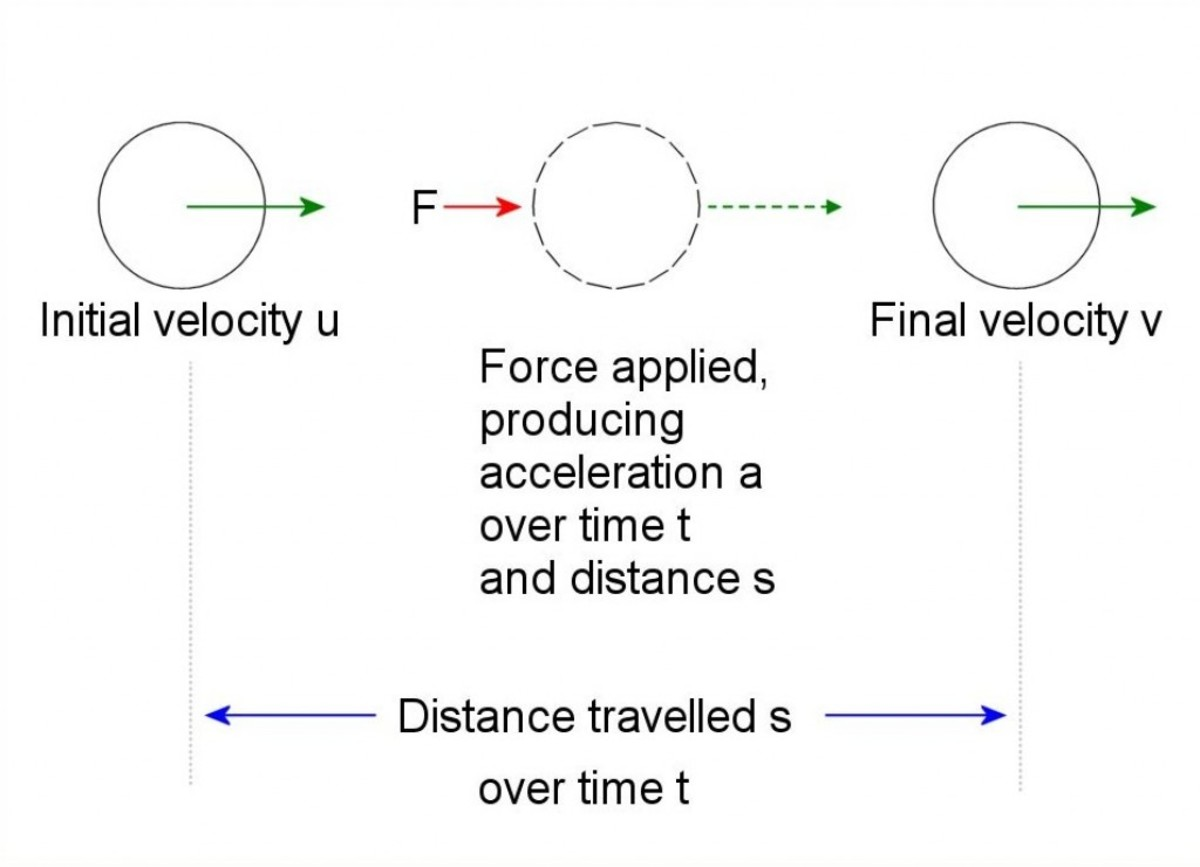Acceleration of body. Force applied produces acceleration a over time t and distance s. © Eugene Brennan

So for uniform acceleration we have three equations:

v = u + at

s = ut + 1/2 at2

v2 = u2 + 2as

Examples:

(1) A force of 100 newtons accelerates a mass of 5 kg for 10 seconds. If the mass is initially at rest, calculate the final velocity.

Firstly it is necessary to calculate the acceleration.

F = 100 newtons

m = 5 kg

F = ma so a = F/m = 100/5 = 20 m/s2

Next work out the final velocity, knowing the acceleration:

Substitute for u, a and t:

u = initial velocity = 0 since the object is at rest

a = 20 m/s2

t = 10 s

v = u + at = 0 + 20 x 10 = 200 m/s

(2) A mass of 10 kg is dropped from the top of a building which is 100 metres tall. How long does it take to reach the ground?

In this example, in theory it doesn't make any difference what the value for the mass is, the acceleration due to gravity is g irrespective of the mass. Galileo demonstrated this when he dropped two balls of equal sizes but differing masses from the leaning tower of Pisa. However in reality, the drag force due to air resistance will slow down the falling mass, so a 10 kg sheet of timber would fall slower than a 10 kg lead weight. So assume there is no drag and the calculations apply to a weight falling in a vacuum.

We know s = 100 m

g = 9.81 m/s2

u = 0 m/s

We can use the equation s = ut + 1/2 at2

The acceleration a in this case is the acceleration due to gravity so a = g

Therefore substituting for u and g gives

s = 0t +1/2 gt2 s = 1/2gt2

Rearranging

t = √(2s/g) = √(2 x 100) / 9.81 = 4.5 seconds approx

## Commander David Scott's Hammmer and Feather Experiment from Apollo 15

### Mechanics

Applied Mechanics by John Hanah and MJ Hillier is a standard text book for students taking Diploma and Technician courses in engineering. It covers the basic concepts I briefly cover in this article as well as as other topics such as motion in a circle, periodic motion, statics and frameworks, impulse and momentum, stress and strain, bending of beams and fluid dynamics. Worked examples are included in each chapter in addition to set problems with answers provided.

### Mathematics

Engineering Mathematics by K.A. Stroud is an excellent math textbook for both engineering students and anyone with an interest in the subject. The material has been written for part 1 of BSc. Engineering Degrees and Higher National Diploma courses.

A wide range of topics are covered including matrices, vectors, complex numbers, calculus, calculus applications, differential equations, series, probability theory, and statistics. The text is written in the style of a personal tutor, guiding the reader through the content, posing questions, and encouraging them to provide the answer.

This book basically makes learning mathematics fun!

## What is Momentum?

Momentum is the product of mass and velocity of a body.

If m is the mass of a body and v is its velocity, then:

momentum = mv

In a collision between two or more bodies, momentum is always conserved. This means that the total momentum of the bodies before the collision equals the total momentum of the bodies after the collision.

So if m1 and m2 are two bodies with velocities of u1 and u2 respectively before the collision and velocities of v1 and v2 after the collision, then:

m1u1 + m2u2 = m1v1 + m2v2

Example:

Two bodies with mass 5 kg and 2 kg and velocities 6 m/s and 3 m/s respectively collide. After the collision the bodies remain joined. Find the velocity of the combined mass.

Let m1 = 5 kg

Let m2 = 2 kg

Let u1 = 6 m/s

Let u2 = 3 m/s

m1u1 + m2u2 = m1v1 + m2v2

Since the bodies are combined after the collision, v1 = v2. Let's call this velocity v.

So:

m1u1 + m2u2 = m1v1 + m2v2 = m1v+ m2v = (m1 + m2)v

Substituting:

(5)(6) + (2)(3) = (5 + 2)v

30 + 6 = 7v

So v = 36/7

## What is Work?

The definition of work in physics is that "work is done when a force moves a body through a distance". If there is no movement of the point of application of a force, no work is done. So for instance, a crane that is simply holding a load at the end of its steel rope is not doing work. Once it starts hoisting the load, it is then doing work. When work is done there is energy transfer. In the crane example, mechanical energy is transferred from the crane to the load, which gains potential energy because of its height above the ground.

The unit of work is the joule.

If work done is W

distance is s

and the force applied is F

then

W = FsCos Θ where Θ is the angle between the force and displacement

Example:

A force F = 50 N is applied to a box of mass 4 kg resting on the ground. Friction between ground and box results in a force opposing motion which is Fₛ = 2 N. Calculate the acceleration and work done sliding the box 3 m.

Start by writing the force equation. Use Newton’s Second Law. The sum of forces produces a net acceleration.

We can use a convention for writing the force equation. So consider the forces acting “forwards” and accelerating the box as positive and forces acting to decelerate the box as negative, Since the friction force Fₛ acts opposite to the direction of motion, its sign is negative, so:

F + (- Fₛ ) = ma

Substituting for F and Fₛ in the first equation gives:

50 + (- 2) = 50 - 2 = 4 x a

Rearranging:

a = (50–2) / 4 = 12 m/s²

Work done on the box is the applied force multiplied by the distance. Work is done opposing friction plus accelerating the box. The net force accelerating force is 50 - 2 = 48 N. The displacement is in the direction of the force, so Θ = 0 and Cos Θ = 1, so:

Work done = FCos Θs = 50 x 1 x 3 = 150 joules

## What is Kinetic Energy?

A body has kinetic energy when it is in motion.

If a body of mass m is moving at a velocity v, then:

Kinetic energy = (1/2)mv2

The kinetic energy of a body at velocity v is the work that must be done on the body to accelerate it to that velocity.

Example:

A rifle bullet of mass 4 grams is moving at a velocity of 1200 m/s.
Calculate its energy.

First convert to SI units, so 4 g = 0.004 kg

Kinetic energy = (1/2)mv2 = (1/2)0.004(1200)2 = 2880 joules

To put this into perspective, this is approximately the same impact energy that a 34kg (75 pound) weight would produce if dropped from chimney height off a two storey house. The bullet has a small mass, but the squared term in the equation increases the energy massively. Every time velocity is doubled, energy increases fourfold. If velocity is tripled, energy increases ninefold.

## What Are Moments, Couples and Torque?

When a force acts on an object, it produces what is known as a turning moment or just simply a moment. An example is when you push on the trunk of a small tree. This produces a turning moment about the base of the tree which is balanced by the tension in the trunk and the restraining force of the roots. If you push too hard, you exceed a breaking limit and the trunk snaps or the tree gets uprooted. The moment of a force about a point is the magnitude of the force multiplied by the perpendicular distance between the force and the point. When 2 forces act in opposite directions, this is known as a couple and the magnitude of the twisting force or couple is called torque. If the forces are both of magnitude F, and the perpendicular distance between them is d, then:

Torque T = Fd

As you can see, if the force is increased or the distance is increased, the torque becomes greater. So this is why it is easier to turn something if it has a larger diameter handle or knob. A tool such as a socket wrench with a longer handle has more torque.

### What is a gearbox used for?

A gearbox is a device which converts high-speed low torque to lower speed and higher torque (or vice versa). Gearboxes are used in vehicles to provide the initial high torque required to get a vehicle moving and accelerate it. Without a gearbox, a much higher powered engine with a resulting higher torque would be needed. Once the vehicle has reached cruising speed, lower torque is required (just sufficient to create the force required to overcome the force of drag and rolling friction at the road surface).

Gearboxes are used in a variety of other applications including power drills, cement mixers (low speed and high torque to turn the drum), food processors and windmills (converting low blade speed to high rotational speed in the generator)

A common misconception is that torque is equivalent to power and more torque equals more power. Remember however torque is a turning force and a gearbox which produces higher torque also reduces speed proportionately. So the power output from a gearbox is equal to the power in (actually a little less because of friction losses, mechanical energy being wasted as heat)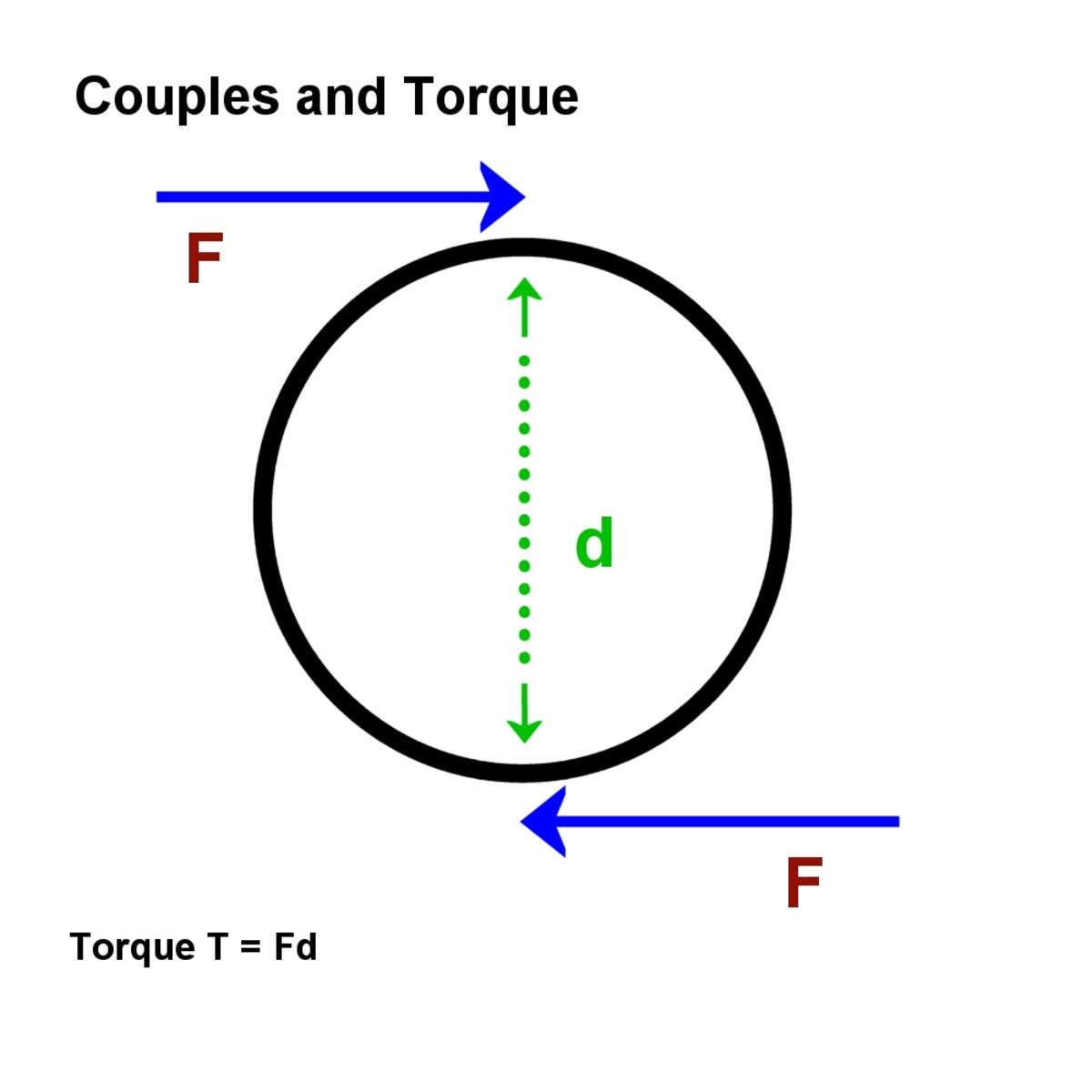Two forces constitute a couple. The magnitude is the torque© Eugene Brennan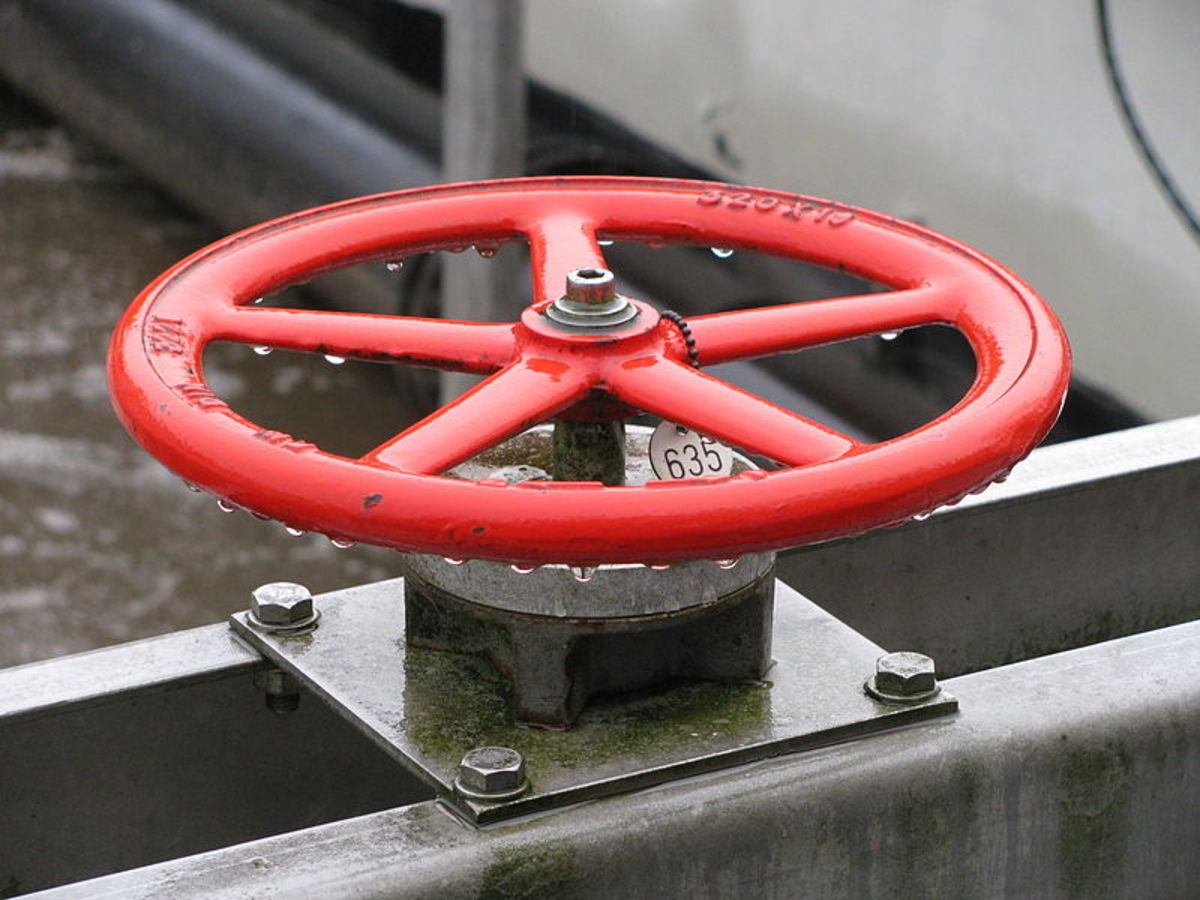This gate valve has a large diameter turning handle to increase torque and make turning of the valve stem easierANKAWÜ, CC by SA via Wikimedia Commons

## Measurement of Angles in Degrees and Radians

Angles are measured in degrees, but sometimes to make the mathematics simpler and elegant it's better to use radians which is another way of denoting an angle. A radian is the angle subtended by an arc of length equal to the radius of the circle. Basically "subtended" is a fancy way of saying that if you draw a line from both ends of the arc to the centre of the circle, this produces an angle with magnitude of 1 radian.

An arc length r corresponds to an angle of 1 radian

So if the circumference of a circle is 2πr = 2π (r) the angle for a full circle is 2π

And 360 degrees = 2π radians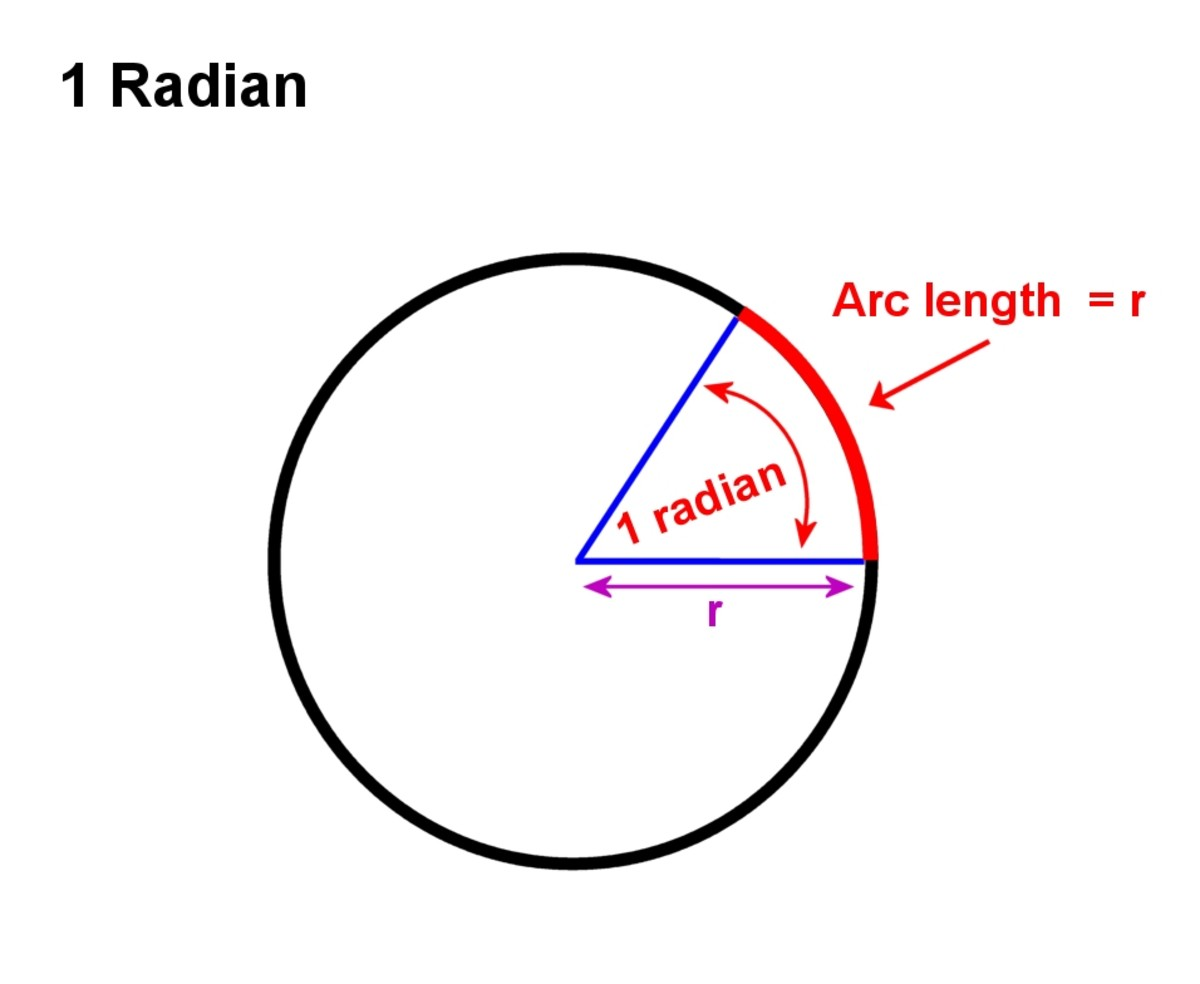1 radian is the angle subtended by an arc of length equal to the radius r© Eugene Brennan

## Angular Velocity

Angular velocity is the speed of rotation of an object. Angular velocity in the "real world" is normally quoted in revolutions per minute (RPM), but it's easier to work with radians and angular velocity in radians per second so that the mathematical equations turn out simpler and more elegant. Angular velocity denoted by the Greek letter ω is the angle in radians that an object rotates through per second.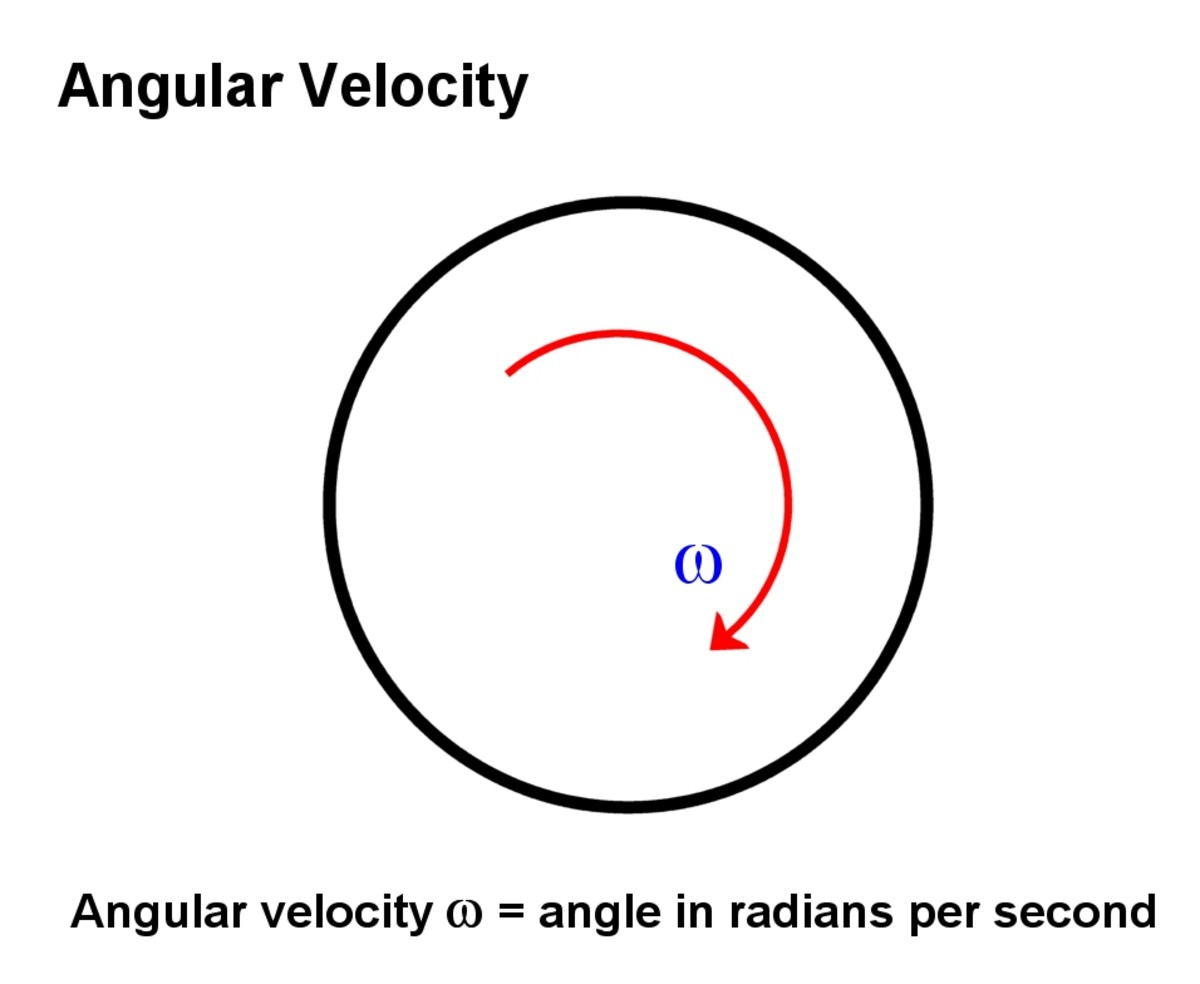Angular velocity denoted by the Greek letter omega, is the angle in radians turned through per second© Eugene Brennan

## What is the Relationship Between Angular Velocity, Torque and Power?

If the angular velocity is ω

and torque is T

Then

Power = ωT

Example:

A shaft from an engine drives a generator at 1000 RPM
The torque produced by the shaft is 1000 Nm

How much mechanical power does the shaft produce at the input to the generator?

1 RPM corresponds to a speed of 1/ 60 RPS (revs per second)
Each revolution corresponds to an angle of 2π radians
So 1 RPM = 2π/60 radians per second
And 1000 RPM = 1000 (2π/60) radians per second

So ω = 1000 (2π/60) = 200π/6 radians per second

Torque T = 1000 Nm

So power = ωT = 200π/6 x 1000 = 104.72 kW

Hannah, J. and Hillerr, M. J., (1971) Applied Mechanics (First metric ed. 1971) Pitman Books Ltd., London, England.

If you liked this article, you may be interested in reading more articles about mechanics:

Solving Projectile Motion Problems - Applying Newton's Equations of Motion to Ballistics

How Do Wheels Work? - The Mechanics of Axles and Wheels

This article is accurate and true to the best of the author’s knowledge. Content is for informational or entertainment purposes only and does not substitute for personal counsel or professional advice in business, financial, legal, or technical matters.

## Questions & Answers

Question: A bowling ball rolled with a force of 15 N accelerates at a rate of 3 m/s²; a second ball rolled with the same force accelerates 4 m/s². What are the masses of the two balls?

Answer: F = ma

So m = F/a

For the first ball

F = 15N

a = 3 m/s²

So

m = F/a = 15/3 = 5 kg

For the second ball

F = 15 N

a = 4 m/s²

So

m = 15/4 = 3.75 kg

Question: How do I calculate the magnitude of force when the amount of force is not given?

Answer: In that case, you would need info about acceleration/deceleration and mass and the time over which it occurs.

Question: A ball is thrown vertically upward from the ground with a speed of 25.5m/s. How long does it take to reach its highest point?

Answer: My other article "Solving Projectile Motion Problems" deals with these sorts of problems. Check it out here:

https://owlcation.com/stem/Solving-Projectile-Moti...

Question: If an object slows from 75 m/s to 3 m/s in 4 secs what is the object's acceleration?

Answer: We know that v = u + at

Where

u is initial velocity

v is final velocity

a is acceleration

t is the time over which acceleration occurs

So

u = 75 m/s

v = 3 m/s

t = 4 secs

v = u + at

Rearranging

a = (v - u)/t

= (3 - 75)/4

= -72/4

= -18 m/s² which is a negative acceleration or deceleration

Question: Calculate when a dock worker applies a constant horizontal force of 80.0 Newton to a block of ice on a smooth horizontal floor. If the frictional force is negligible, the block starts from rest and moves 11.0 meters in 5 seconds (a) What is the mass of the block of ice?(b) If the worker stops pushing at the end of 5 seconds, how far does the block move in the next 5 seconds?

Newton's 2nd Law

F = ma

Since there's no opposing force on the block of ice, the net force on the block is F = 80N

So 80 = ma or m = 80/a

To find m, we need to find a

Using Newton's equations of motion:

Initial velocity u = 0

Distance s = 11m

Time t = 5 seconds

Use s = ut + 1/2 at² because it's the only equation which gives us the acceleration a, while knowing all the other variables.

Substituting gives:

11 = (0)(5) + 1/2a(5²)

Rearranging:

11 = (1/2)a(25)

So:

a = 22/25 m/s²

Substituting in the equation m = 80/a gives:

m = 80 / (22/25) or m = 90.9 kg approx

(b)

Since there's no further acceleration (the worker stops pushing), and there's no deceleration (friction is negligible), the block will move at constant velocity (Newton's first law of motion).

So:

Use s = ut + 1/2 at² again

Since a = 0

s = ut + 1/2 (0)t²

or

s = ut

But we don't know the initial velocity u that the block travels at after the worker stops pushing. So first we have to go back and find it using the first equation of motion. We need to find v the final velocity after pushing and this will become the initial velocity u after pushing stops:

v = u + at

Substituting gives:

v = 0 + at = 0 + (22/25)5 = 110/25 = 22/5 m/s

So after the worker stops pushing

V = 22/5 m/s so u = 22/5 m/s

t = 5 s

a = 0 m/s²

Now substitute into s = ut + 1/2 at²

s = (22/5)(5) + (1/2)(0)(5² )

Or s = 22 m

Question: What is the magnitude of the friction between the wheels and the ground?

Answer: Friction is necessary between wheels and the ground in order to prevent the wheels slipping. Static friction doesn't oppose motion, but rolling friction can do so.

In the case of a wheel driving a vehicle, if the driving torque of the wheel turning clockwise is T and the wheel's radius is r, this results in a couple. So there's a force at the point of contact of the wheel and ground of F = T/r acting backwards and F = T/r acting forwards on the axle. If there's no slippage, a balancing force F = T/R acts forwards at the point of contact on the ground. So these forces are in balance. The other unbalanced force at the axle pushes the vehicle forwards.

Question: If a force of 10N acts on a body of weight 20N at rest, what is the velocity?

Answer: The velocity depends on how long the force acts for.

Since the weight is 20N and weight = mg where g is the acceleration due to gravity:

Then

g = 9.81

mg = 20

So m = 20/g = 20/9.81

We know F = ma

So a = F/m

v = u + at

So

v = u + (F/m)t

Substituting

u = 0

m = 20/9.81

F = 10

So

v = 0 + (10/(20/9.81))t

= 4.905t m/s where t is in seconds

This result is for when the body is in free space and neglects the effects of friction (e.g., if the body is resting on a surface). Friction opposes the accelerating force and results in a lower net force on the body.

Question: A spring stretches by 6cm when supporting a load of 15N. By how much would it stretch when supporting a load of 5kg?

Answer: The extension is proportional to the tension in the spring (Hooke's Law)

So if F is the applied force , x is the extension and k is the spring constant

F = kx

or k = F/x

Plugging in the values

k = 15/6 N/cm

For a weight of 5 kg

F = mg

m = 5 kg

g = 9.81

So F = 5 x 9.81 = 49.05 N

Since F = kx for the spring

Rearranging:

x = F/k

Substituting values:

x = 49.05 / (15/6) = 19.62 cm

Question: What is the difference between torque and moments because both of them are calculated the same way?

Answer: A moment is the product of a single force about a point. E.g. when you push down on the end of a wheel brace on a nut on a car wheel.

A couple is two forces acting together, and the magnitude is the torque.

In the wheel brace example, the force produces both a couple (whose magnitude is the torque) and a force at the nut (which pushes the nut).

In a sense, they are the same, but there are subtle differences.

Have a look at this discussion:

https://www.quora.com/What-is-the-difference-betwe...

Question: A metal ball is dropped from the roof of a building 75m high. Neglecting air resistance, what is the velocity of the ball five seconds before it reaches the ground?

Answer: V^2 = u^2 + 2as can't be used because s is unknown.

How about v = u +at ?

t is unknown, but if you could find t when the ball hits the ground, you could just subtract 5 seconds from it and use it in the above equation.

So use s = ut + 1/2at^2

u = 0

a = g = 9.81 m/s^2

s = 75 m

So

s = ut + 1/2at^2

But u = 0

So

s = 1/2at^2

and

t = t = square root(2h/g)

Substituting

t = t = square root(2(75)/9.81) = 3.91 seconds

So 5 seconds before the ball hits the ground, the velocity of the ball is zero because it hasn't been released!

For more info on projectile motion and the equations for objects dropped, thrown up or projected at an angle from the ground, see my other tutorial:

https://owlcation.com/stem/Solving-Projectile-Moti...

Question: If a 2000kg satellite orbits around the earth at the height of 300km, what is the speed of the satellite and its period?

Answer: The orbital speed is independent of the mass of the satellite if the mass is much less than that of the Earth so.

The equation for orbital velocity is v = Square Root (GM / r)

Where v is the linear velocity

G is the gravitational constant = 6.674×10^-11 m^3kg^-1s^-2

M is the mass of the Earth = 5.9722 × 10^24 kg

and r is the distance from the Earth to the satellite = 300 x 10^6 metres

Also v = rw = but w = 2PI/T

where w is the angular velocity

and T is the period of orbit,

So substituting gives

v = r(2PI/T)

And rearranging

T = r2PI/T or T = 2PIr/v

substitute the values r = 300 x 10^6 and v calculated previously to get T

Question: What is the proofing of the Galilean invariance?

Answer: Have a look at this link, it will probably be helpful:

Question: Assuming that the Earth's moon is at an instance 382,000,000m away from the centre of the earth, what are its linear speed and the period of orbit at motion around the earth?

Answer: The equation for orbital velocity is v = Square Root (GM / r)

Where v is the linear velocity

G is the gravitational constant

M is the mass of the Earth

and r is the distance from the Earth to the satellite (the Moon in this case) = 382 x 10^6 metres

So look up values for G & M, plug them into the equation you'll get an answer.

Also v = rw = but w = 2PI/T

where w is the angular velocity

and T is the period of orbit,

So substituting gives

v = r(2PI/T)

And rearranging

T = r2PI/T or T = 2PIr/v

substitute the values r = 382 x 10^6 and v calculated previously to get T

Question: A 1.5kg mass is moving in a circular motion with a radius 0.8m. If the stone moves with a constant speed of 4.0m/s, what is the maximum and minimum tension on the string?

Answer: The centripetal force on the stone is provided by the tension in the string.

Its magnitude is F = mv^2/r

Where m is the mass = 1.5 kg

v is the linear velocity of the stone = 4.0 m/s

and r is the radius of curvature = 0.8 m

So F = (1.5)(4.0^2)/0.8 = 19.2 N

Question: An electrically driven crane rises a load of mass 238 kg from the ground, accelerating it from rest to a speed of v = 0.8 m/s over a distance of h =5 m. Friction resistance to motion is Ff = 113 N.

a) What is the work input from the driving motor?

b) What is the tension in the lifting cable?

c) What is the maximum power developed by the driving motor?

Answer: The weight of the load mg acts downwards.

Assume a force F exerted by the rope which accelerates the mass, acts upwards.

The sum of the forces acting on a mass equals the mass x acceleration. (Newton's second law)

Assume forces in the upwards direction are positive, so the force equation is:

F - mg - Ff = ma

(Because the force upwards minus the force due to weight downwards minus the friction force = ma. It's the net force that accelerates the mass. In this case, the crane has to overcome both the friction force and the weight of the mass. It's "what's left over" that does the acceleration)

So we need to find F and a.

We can find a using the equations of motion.

We know initial velocity u = 0 m/s

Final velocity v = 0.8 m/s

Distance s = h = 5 m

Ff = 113 N

m = 238 kg

g = 9.81 m/s²

The equation to use is:

v² = u² + 2as

Substituting:

0.8² = 0² + 2a5

Rearranging:

a = 0.8²/(2 x 5) = 0.064 m/s²

Substituting in F - mg - Ff = ma gives

F - 238 x 9.81 - 113 = 238 x 0.064

Rearranging:

F = 238 x 0.064 + 238 x 9.81 + 113 = 2463 N

a) Work input = Force x distance = 2463 x 5 = 12,315 joules

This has three components:

Work done overcoming friction.

Work done overcoming the weight of the load

Work done accelerating load

b) Tension in cable equals the lifting force = 2463 N

c) Max power input = Force x distance / time taken = Force x final velocity

= 2463 x 5 = 13.315 kw

Work input is the energy used. The definition of work is that "work is done when a force moves a body through a distance." So work is Fs where F is the force and s is the distance.

I think all this is correct; if you have answers, you can check calculations.

© 2012 Eugene Brennan

ellery on February 26, 2020:

If a question asked; one end of a cord is fixed and a small 0.500Kg object is attacted to the other end, where it swings in a section of a vertical circle of radius 2.00m. When the angle = 20.0 degree, the speed of the oject is 8 m/s. At this instant, find (a) the tention in the string, (b) the tanggential and the radius components of the acceleration, and (c) the total acceleration.

Eugene Brennan (author) from Ireland on February 10, 2020:

Hi Chris. Newtons is a measure of force. Knowing the force and mass, you work out acceleration using the equation a = F/m. Then use the equations of motion to work out velocity v in metres per second and convert that to mph.

Chris on February 10, 2020:

How do I convert Newtons to MPH?

ukohemmaelnu on January 15, 2020:

thank you sir

Johannes L Strumpfer on November 28, 2019:

Thanx a lot for the help.

I'm going to have to sit down and really focus on the math behind just the basic forces involved first. I understand the mechanics of forces and how to manipulate them but my maths ability needs some work. The basic idea behind this project is to use leverage from 12 or so levels of 4 x levers which is driven by electro magnets pushing permanent magnets which is at the ends of the levers/arms. All levels are attached to a verical axle via either a freewheel or clutch system. At the end of the axle there is a heavy weight also attached via a clutch system(or not still working on it). All level's levers/arms has weights that can slide out and in along the arm/levers. The weights are for leverage. When the system starts up at first, all the levels will work together to get momentum going(weights are at max radius). And once a good speed is reached the system will switch between leverage and velocity systematicly bringing the weights in one after the other (just like an ice skater going into a spin) and then out again as velocity slows down, to be propelled by the electro magnets again. This is one half of the machine.

Eugene Brennan (author) from Ireland on November 26, 2019:

It's sort of out of my depth and I haven't studied this stuff for over 30 years, but maybe try and understand moment of inertia and angular momentum. Things are simple in 2D on a plane but become more complicated in three dimensions. Linear momentum is just mv where m is mass and v is velocity. For rotating objects in 2D, the equivalent is L (angular monmentum) = Iw where I is the moment of inertia and w is the angular velocity. Similarly a force F = ma for linear motion and for rotational motion, T = Iα where T is torque and α is angular acceleration. That's for mass concentrated at a point, but it becomes more complicated if the shape of things is different. Moment of inertia changes when the shape of an object varies even though the mass is the same (ballet dancers bringing their arms in to their side reduces their moment of inertia, and since angular momentum is conserved, angular velocity increases).

Johannes L Strumpfer on November 26, 2019:

Wowzer. Going to take me a while to work through. Thanks though.

Eugene Brennan (author) from Ireland on November 26, 2019:

Have a look at this Wikipedia article which discusses angular momentum.

https://en.wikipedia.org/wiki/Angular_momentum

Johannes L Strumpfer on November 26, 2019:

1. How long would 4 levers conected to a single axle have to be to easily get 500kg to accelerate. The weight is at the end of the axle

2. How much magnetic force would that require if at the ends of the levers there are permanent magnets driven by electro magnets.

3. Say that there are also weights next to the permanent magnets which is capable of moving in and out by sliding on the levers what effect would this have on acceleration, momentum and velocity?

If you would like a better picture of what I have been working on I can draw it so you can have a clearer picture of all the forces and mechanics it deals with. It has alot to do with rotational force which changes from a wide diameter to provide leverage and then into a small diameter to increase velocity. Similar to what and ice scater would do to go into a spin.

Eugene Brennan (author) from Ireland on November 25, 2019:

Johann on November 25, 2019:

Hi Eugene Brennan,

I have a few physics questions regarding a project I've been working on for quite a long time. Would you mind helping a simpleton like me out to try and see if I could get it to work?

Eugene Brennan (author) from Ireland on October 12, 2019:

If the velocity changes by 20 m/s in 2 minutes, that's an acceleration of 20/120 seconds = 1/6 m/s/s.

F = ma = 100 kg x 1/6 = 16 2/3 Newtons

Gloria on October 12, 2019:

A body of mass 100kg moves with a velocity of 20ms^-1 in 2minutes find the forces of the body

usman jallow on May 08, 2019:

I am very greatful..

Thankz guyz

agboola taiwo on March 27, 2019:

thanks for the help

Eugene Brennan (author) from Ireland on March 22, 2019:

Esther's question.

Let v1 be the velocity before impact and v2 the velocity after impact.

m1 is the weight of the hammer = 1.1 tonne = 1100kg

m2 is the weight of the pile = 120kg

h = 750mm = 0.75m

Kinetic energy before impact = potential energy above the pile.

So ½m1v1² = m1gh

m1 cancels out

Rearranging gives v1 = √(2gh).

(Note: You could also have worked out v1 from the equation of motion v² = u² + 2as where a = g and s = the height h and initial velocity = 0 m/s)

Substituting gives v1 = √(2 x 9.81 x 0.75) =3.84 m/s

Nextuse use conservation of momentum

Momentum of pile and hammer after impact = momentum of hammer before impact

So (120 + 1100)v2 = 1100 x 3.84

Rearranging gives v2 = 3.46 m/s

The distance 150mm isn't used in the above calculations, but you can use it to find the resisting force of the ground bring the pile to rest.

Eugene Brennan (author) from Ireland on March 22, 2019:

Heirnie's question first.

Let the masses be m1 and m2 and the force be F and the acceleration a1 and after the second mass added a2

So F = m1a1

But when the new mass is added acceleration becomes 1/3 of what it was previously so a2 = a1/3

So F = (m1 + m2)a2 = (m1 + m2)a1/3

Since F is the same in both cases

m1a1 = (m1 + m2)a1/3

so m1 = (m1 + m2)/3

or 3m1 = m1 + m2

So m2 = 2m1

The second mass is twice the first mass.

esther on March 21, 2019:

how do i calculate this .a vertical pile of mass 120 kg is driven 150 mm into the ground b the blow of a 1.1 t hammer which falls through 750 mm assumming it does not bounce the velocity after the impact is?

Heirnie on March 04, 2019:

A net force acts on mass and creates an acceleration. A mass is added to mass . The same net force acting on the two masses together creates one-third the acceleration. Determine the ratio .

Eugene Brennan (author) from Ireland on May 24, 2018:

Here's some more definitions on displacement and tonnage:

http://www.themaritimesite.com/a-guide-to-understa...

Eugene Brennan (author) from Ireland on May 07, 2018:

Hi Cheyenne, look in my profile, there's several more!

Cheyenne Leo from British Columbia, Canada on May 07, 2018:

This is fantastic. Do you have any more of these sorts of articles, educational in nature?

Eugene Brennan (author) from Ireland on December 07, 2017:

Hi Diego,

No, I define the reactive force as being a reaction to the active force. So for instance a spring "pushes" back when it is compressed. The reaction produced by the object in question doesn't act on itself, it acts on whatever produced the force. Which part of the text or graphics do you think is misleading?

Eugene Brennan (author) from Ireland on June 10, 2017:

A Newton's cradle is a device which demonstrates how impact energy can be transferred using a series of swinging spheres. The first swinging sphere collides with the second sphere and because the collision is elastic, it slows down and transfers most of its kinetic energy to the second sphere which then gains momentum and hence velocity. This sphere collides with the third sphere and the process is repeated, energy being transferred through the series of balls.

Jovito B. Catungal on June 09, 2017:

This is good review material.

I have a queryy.. Is there a gadget to convert the impact energy or a force into a velocity?

Akinmolujoye Abiodun on June 02, 2017:

I'm very grateful thanks guy

Eugene Brennan (author) from Ireland on June 06, 2015:

Thanks Jackie, glad you liked it!

John Jack George from United States on June 05, 2015:

Awesome Hub about Physics! Thanks for all the great info!